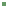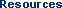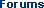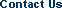Log InRegister
 Notes from South Mountain

How to Use Herb Concentrates

by Andrew Ellis

Prescribing and dosing granular herb concentrates requires a slightly different reasoning process and different mathematics from those used for crude herbs for 2 reasons. First, the concentrated herb granules are available in both formula and single-herb form. Thus, one must consider how to combine and dose these two forms. Second, experience has shown that for herb concentrates, 10 grams per day (approximately three grams, three times a day) is generally an effective dose. Thus, the prescribed herbs and formulas must be fit into a 10-grams-per-day dose.

When prescribing an unmodified an unmodified formula, dosing 10 grams per day is simple and straightforward. However, if more than one formula is used, or if single herbs are added to the prescription, there are three possible strategies:

1. One or Two formulas, with or without single herbs.
2. Three or more formulas, with or without single herbs.
3. Combining only single herbs.

We discuss each of these strategies in the section following including case studies.

One or Two Formulas with or without Single Herbs

This strategy uses one or two classical formulas as the base for treatment and adds single herbs to meet the exact needs of the patient or offset an aspect of the base formula that is contrary to the needs of patient. This is the most common way of prescribing herb concentrates.

For example:

A patient suffers from blood vacuity as evidenced by scant menstrual flow, pale tongue, fine pulse and dizziness. She complains of restless sleep and waking during the night. The prescription aims to treat blood vacuity with the classic formula for that pattern, Si Wu Tang (Tangkuei Four Combination). Herbs are added to address the patient's specific sleep disorders. A ten-day formula is prescribed.

 • Si Wu Tang 75 grams • Ye Jiao Teng (Polygonum multifloru Vine) 15 grams • Suan Zao Ren (Zizyphus) 10 grams Total 100 grams

A six-year-old boy presents with chronic nasal congestion. The practitioner determines from the boy's pulse, tongue, and other signs and symptoms that his constitution is one that would benefit from Bu Zhong Yi Qi Tang ( Ginseng & Astragalus Combination ). Because the boy's nasal discharge is slightly yellow, Cang Er San (Xanthium Formula and Huang Qin (Scute) are added to address branch symptoms. A ten-day formula is prescribed as follows (2 grams, three imes per day is a typical child's dose):

 • Bu Zhong Yi Qi Tang 25 grams • Cang Er San 30 grams • Huang Qin ( Scute ) 5 grams Total 60 grams

After ten days the discharge has decreased markedly and the following ten- day formula is prescribed:

 • Bu Zhong Yi Qi Tang 35 grams • Cang Er San 20 grams • Huang Qin ( Scute ) 5 grams Total 60 grams

This formula modification shows that the treatment principle is shifted from one that emphasizes the branch disorder to one that emphasizes the root constitutional imbalance. By utilizing one formula for root and one for branch it is relatively simple to implement a gradual shift from treatment of branch to treatment of root as symptoms improve.

Three or More Formulas with or without Single Herbs

This method is most frequently used to treat a complex disorder for which a classic formula is unavailable. In the following example we take the same six-year-old boy as in the previous example. In this case, however, he also presents with heat at night, restless sleep (with tossing and turning and discarding of blankets), thirst, and a fine, rapid pulse. Thus, the practitioner wishes to nourish yin and reduce the drying nature of the formula. This can be accomplished by adding Liu Wei Di Huang Wan ( Rehmannia Six Formula ) to the above prescription and making other modifications. The ten-day formula is as follows ( 2 grams, three times per day is a typical child's dose):

 • Bu Zhong Yi Qi Tang 15 grams • Cang Er San 7 grams • Huang Qin 5 grams • Liu Wei Di Huang Wan 15 grams • Qing Bi Tang ( Pueraria Nasal Combination ) 8 grams • Shi Hu (Ephemetantha Fimbriata) 5 grams • Ge Gen ( Pueraria Root ) 5 grams Total 60 grams

In this case, the dose of Cang Er San is reduced because it is considered too drying. The treatment of the branch symptom of nasal congestion is amended by including Qing Bi Tang (Pueraria Nasal Combination), which is less damaging to the yin and fluids than Cang Er San. Agents to address yin-fluid vacuity and thirst are also added.

Combination of Single Herbs Only

This strategy is employed when no formula can be found that meets the patient's disease pattern or when one needs to limit the number of herbs used, for instance, to determine if a patient has a reaction to specific herbs. It is best to keep the number of herbs in the formula to below ten so that enough of each herb can be put into a 10-gran-per-day dose. An example of this type of formula follows:

A fifty-year-old woman is diagnosed with heart disease by her Western medical practitioner. She presents with chest pain, bouts of weakness, and dizziness and shortness of breath. Chinese medicine sees this as a combination of blood stasis and qi vacuity. The following formula is prescribed:

 • San Qi (Pseudoginseng) 15 grams • Dan Shen (Salvia) 20 grams • Ren Shen (Ginseng) 17 grams • Xue Jie (Dragon's Blood) 8 grams Total 60 grams

Since this formula consists of only four herbs, it is not necessary to prescribe 10 grams per day. In this case, 2 grams per dose, three times per day is sufficient. Note that the amount of Xue Jie (Dragon's Blood) is kept small because that herb is difficult to digest.

Dosage Determination

Concentrates are vacuum-dried granules of a decocted herb or formula. As discussed in the Introduction, the efficient extraction process used in producing concentrates differs considerably from the process of decocting crude herbs in the patient's home. Thus, calculating the dosage of herb concentrates demands a unique method, quite unlike that used for crude herbs. The system described here is based on observation of prescription methods in Taiwan, where concentrated granules have long been an accepted form of herbal therapy.

The usual adult dose is 3 grams, three times a day. To simplify calculations one can round this off to 10 grams per day. Appropriate dosages of each component of combinations, such as those discussed above, can be determined through a four-step process.

Step 1. Calculate the total number of grams to be dispensed by multiplying the grams per day times the number of days the formula will be taken. For example, if 10 grams of herbs per day are to be prescribed for a ten-day period, the total amount of herbs to be dispensed is 100 grams.

Step 2. Dose the single herbs in the prescription at 0.5 to 2 grams/day. Herbs typically given in small dose such as Yuan Zhi (Polygala) and Fu Zi (Aconite) should be dosed at about 0.5 grams per day; herbs which are typically prescribed in large doses such as Ye Jiao Teng (Polygonum multifloru Vine) and Yi Yi Ren (Coix) should be prescribed at 1.5 to 2 grams per day. Most herbs should be dosed at about 1 gram per day.

Step 3. After dosing the single herbs, find the sum of all single-herb dosages and deduct that number from the total grams to be dispensed as determined in step 1.This is the number of grams left for formulas in the prescription.

Step 4. Divide this amount determined in step 3 among the formulas in the prescription, giving a large amount to the formula or formulas that you wish to emphasize.

To take the formula prescribed in the first strategy discussion above as an example:

 • Si Wu Tang 75 grams • Ye Jiao Teng 15 grams • Suan Zao Ren ( Zizyphus ) 10 grams Total 100 grams

Here is the thinking behind the dosage assignments in this prescription.

Step 1. The prescription is for ten days at is 10 grams per day. Thus, the total number of grams that will be dispensed is 100.

Step 2. The prescription has two single herbs. Since Ye Jiao Teng is commonly given in large dosages (especially when being used to treat insomnia), assign it a dosage of 1.5 grams per day, totaling 15 grams for ten days. Suan Zao Ren ( Zizyphus ) is assigned the normal daily dose for herb concentrates of 1 gram per day totaling 10 grams for ten-days period.

Step 3. The total number of grams assigned to single herbs is 25 (10 for Suan Zao Ren and 15 for Ye Jiao Teng). Subtracting this from the total number of grams to be dispensed (100) leaves 75 grams for formulas.

Step 4. Since there is only one formula in this prescription, all 75 grams are assigned to it. If there is a need for an additional formula, the 75 grams can be divided between the formulas, giving a larger percentage to the formula that needs to be to emphasized.